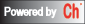# CGI-Based Web Plotter

Note: the function for plotting should be expressed in the syntax of C/Ch. There is no exponential operator in C/Ch. For a mathematical expression x2, use x*x or pow(x, 2), do not use x^2. For an expression x3, use x*x*x or pow(x, 3), do not use x^3.

## 2D Plotter

```
Function: y =
X-min:      X-max:    Number of points:

```

## 3D Plotter

```
Function: z =
X-min:      X-max:    Number of x points:

Y-min:      X-max:    Number of y points:

```

## Interactive Plotting Examples

Enter the desired dimension and number of data sets for your plot.

Dimension of Plot :
Number of Data Sets to Plot :

## Options:

Use Ranges
Use Legends